# Varactor multipliers

Home - Techniek - Electronica - Radiotechniek - Radio amateur bladen - QST - Varactor multipliers

Appreciable power output at 432 Mc. from a device that needs no d.c. power supply and at the same time works at high efficiency would seem to be the answer to a u.h.f. man's prayer - or a pipe dream! It's no dream. The device that does it is the varactor diode.

Here is a group of three articles aimed at giving you background information on the varactor multiplier - what it is, how it works - along with practical construction data on equipment. The leadoff article, by W10ER, is on the varactor and its application. The second, by W1KLK, describes 432-Mc. triplers he and W1CER built, and their experiences in getting them into operation. The third, by WIIGJ, tells how to build a varactor tripler-converter for transferring a 50-Mc. s.s.b. signal to 432-Mc.

On a watts-per-dollar basis, varactor multiplication is a pretty attractive method for moving up in the spectrum!

## Varactor diodes in theory and practice

### A Treatise for the Solid-State Neophyte

Until recent years the u.h.f. enthusiast had little to look forward to as far as low cost was concerned when building a transmitter for use on 420 Mc. Expensive vacuum tubes and related component parts were required to generate power levels as low as 10 watts. With the advent of varactor diodes (variable reactance diodes) many of the problems were resolved. The varactor offers many advantages over vacuum tubes: no power supply is required, doublers and triplers are capable of efficiencies as great as 70 per cent, unit cost is below that of like-power circuits using tubes, the finished product does not generate high amounts of heat, and is capable of being miniaturized in a way that had been previously unheard of.

It became convenient to install a varactor doubler at the output terminal of the 220-Mc. transmitter (tuned down to 216 Mc.) to secure f.m. or c.w. on 432 Mc. A.m. signals could also be multiplied, but with the inherent distortion products brought about by the multiplication of a complex waveform. (Nevertheless, the resultant a.m. signal is still acceptable for voice communications.) In a similar fashion, the output from a 2-meter transmitter could be applied to the input terminals of a varactor tripler for the purpose of securing output at 432 Mc. Thus came the dawning of many new practices - applicable to industry and amateur radio as well.

Varactor diodes have been used successfully in parametric-amplifier circuitry, as mixers for the generation of u.h.f. s.s.b. energy, and as frequency multipliers in solid-state mobile equipment. Although there is a variety of applications useful to radio amateurs in which the varactor diode can be used, this article will deal with their use as frequency doublers and tripiers. The varactor diode used in the tests which made much of this data possible was the Amperex 114A (1N4885).(1) The H4A is designed to handle up to 30 watts of r.f. input power when operating normally. Efficiencies of 60 per cent doubling to 144 Mc. and 50 per cent tripling to 432 Mc. are readily obtainable.

### The Varactor Device

In ordinary terms, when we refer to a semiconductor diode, we think of a 2-terminal p-n junction operated in the forward-conduction region as is the case with a rectifier. Alternatively, we think of the device as operating in the reverse-avalanche region, exemplified by the zener diode. When considering these functions of the more common diodes we might rightly conclude that the term diode is not particularly applicable to varactors, since they operate neither as rectifiers nor as avalanche devices. In reality, varactor diodes operate in the region between forward conduction and reverse breakdown - the region in which a conventional diode is considered to be cut off. In this region the p-n junction can be compared to a capacitor in series with a resistor as illustrated in Fig. 1, at A. The capacitance varies as a nonlinear function of the applied voltage, and it is this nonlinearity that encourages the generation of harmonic energy.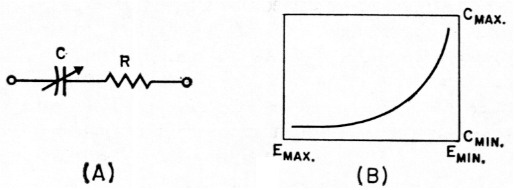Fig. 1. (A) A varactor diode is equivalent to a variable capactor in series with a resistor. (B) Typical voltage-capacitance curve for an abrupt-junction varactor. The capacitance at the varactor junction decreases as the reverse bias voltage is increased.

The component R, in Fig. 1, represents the resistance of the varactor's junction as well as of the bulk material from which the varactor is made. This resistance determines the quality of the varactor, making it necessary during the manufacturing process to hold this value to an absolute minimum. The typical varactor diode has a series resistance of the order of 1 ohm, or less. Although this may seem like a small quantity of resistance, the resultant losses caused by the total current through the device are quite significant.

The "cutoff frequency" (fco) of a given varactor diode can be found by, consulting the manufacturer's specification sheet and will enable the user to compare the merits of the various types when making a selection for a particular frequency of operation. It is best to select a varactor whose A. is much higher than the intended operating frequency, rather to choose one with an f which is quite close to the operating frequency. This is true because as the operating frequency approaches the cutoff frequency, the varactor becomes more "lossy." Varactor manufacturers say that the cutoff frequency is related to the junction capacitance of the diode, and to its series resistance. The cutoff frequency is defined arbitrarily as that frequency at which the varactor's Q equals 1. Using the values of junction capacitance (C) and the series resistance (Rs), given on the varactor specification sheet, fco can be determined from: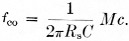### How a Varactor Works

The performance of the varactor's junction capacitance can be understood by reverting to basic semiconductor theory:(2)

When a junction is formed between n-type and p-type material, a cross-migration of charges takes place across the junction of the semiconductor materials (Fig. 2). This means that the electrons from the n-type material flow across the varactor's junction to neutralize the positive carriers near the junction in the p-type material. At the same time, the "holes" from the p-type material cross over the varactor's junction and neutralize the excess electrons near the junction in the n-type material of the diode. Because of this cross-migration process, all of the free charged particles are removed from the immediate vicinity of the junction, creating what is called a "depletion layer" in the junction.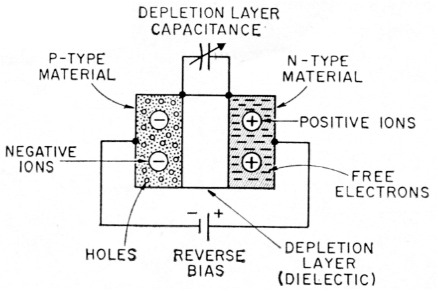Fig. 2. Basic structure of a varactor diode, showing the relationship between the p.n. material and the depletion layer.

The varactor diode performs very much like a lightly-charged capacitor with a relatively high Q factor. The depletion layer represents the dielectric and the semiconductor material on each side of the junction acts as the two plates of the capacitor. If an external voltage is applied across the p-n junction (reverse bias) the depletion layer will widen and the capacitance between the two materials will decrease. Conversely, if a forward voltage is connected across the p-n junction, the depletion layer will become thinner and the capacitance between the two materials will increase. (Bias can be developed across a resistor which is connected in parallel with the varactor. The system is not unlike the grid-leak principle used with vacuum tubes. It is possible to apply a fixed bias to the varactor rather than using the grid-leak method. The latter is not commonly practiced, however.) It is obvious therefore, that the junction capacitance will vary in relation to the externally-applied voltage as long as the junction is reverse-biased. The two conditions, for reverse bias, are low voltage for high capacitance and high voltage for low capacitance. A curve that illustrates this relationship is given in Fig. 1, at B. Generally, the capacitance of the depletion layer is inversely proportional to the square root, or cube root, of the applied voltage, depending on the type of varactor used.

The two most common types in use are the "abrupt-junction" varactor and the "graded-junction" varactor. The significant difference between the two types is that the graded-junction varactor has an impurity content in its semiconductor materials that permits a gradual and linear change from p-type to n-type material while the abrupt-junction varactor has an impurity characteristic, or profile, that results in a rapid change from p-type to n-type material. This article will deal with the more common abrupt-junction varactor which follows the square-root principle.

### A Shunt-Type Frequency Doubler

It can be seen from the foregoing discussion that a condition of reactive nonlinearity exists within the varactor diode when an r.f. signal is applied to it. This reactive nonlinearity, without an appreciable series-resistance component, enables the varactor to generate harmonics very efficiently when used in circuits of the shunt frequency-multiplier variety shown in Fig. 3. With vacuum-tube harmonic generators the amplitude-distortion principle is used. In this case the tube is operated on the nonlinear portion of its curve, resulting in a plate-current change that is not directly proportional to a given change in grid voltage. Under these conditions, the amplitude of the output waveform will be distorted, giving rise to the production of harmonic energy (The Radio Amateur's Handbook, Chapter 3).

By comparison, the parametric-distortion principle is used when generating harmonics with varactor diodes. Harmonic generation is brought about by applying a current through the varactor. The resulting voltage which appears across the terminals of the diode is highly distorted and is, therefore, rich in harmonic energy. This action, plus the variation in capacitance at the varactor junction with r.f. energy applied, enables the varactor to generate harmonics.

The circuit in Fig. 3, a frequency doubler, is driven by a sinusoidal voltage source (V,) having a fundamental frequency f and an internal impedance Z,. Since the input tuned circuit, formed by L1 and C1, presents a reasonably high impedance to all frequencies other than the fundamental, essentially only the fundamental component of current (Ii) can flow in the input loop. A second-harmonic current, 72i, is generated by the varactor diode (CR1) and flows through the load ZL. Another tuned circuit, L2C2, is inserted in the output loop to block the fundamental-frequency energy and is resonant at the desired output frequency.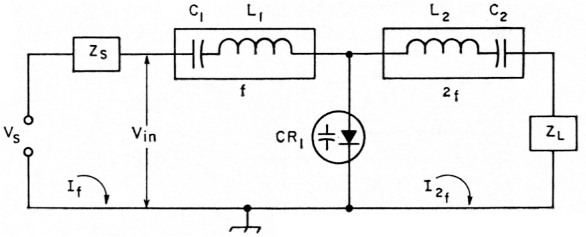Fig. 3. Basic shunt-type frequency doubler.

### The Shunt Frequency Tripler

The popular circuit configuration shown in Fig. 4 illustrates the basic arrangement for a shunt-type tripler. A sinusoidal voltage source (Vs), having an internal impedance Zs, is applied to the input terminals of the circuit. The input network (L1C1) is tuned to the fundamental frequency and presents a reasonably high impedance to all frequencies other than the fundamental. The varactor, having a nonlinear characteristic, generates a second-harmonic current, I2, that will flow through the diode and L2C2. The network L2C2 acts as a high-impedance device to all frequencies other than 2f. The currents I1 and I2 flow through CR1, along with frequencies f and 2f, causing CR1 to function as a mixer, producing a frequency 3f. The remaining tuned circuit, L3C3, acts as a high impedance to all frequencies other than 3f, enabling the third-harmonic energy to flow to ZL. The tuned circuit L2C2 is commonly referred to as the "idler tank".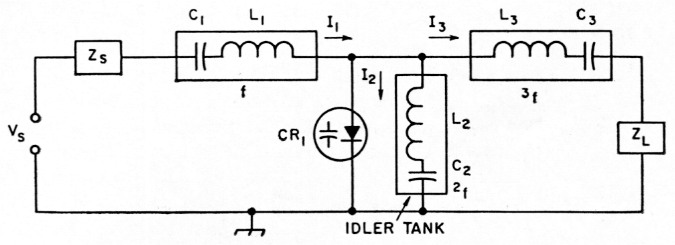Fig. 4. Basic circuit of the shunt-type frequency tripler.

### Some Design Considerations

Varactors can be used as frequency quadrupiers, quintuplera, and as multipliers to frequencies that are even farther removed from the fundamental frequency. It is claimed by some engineers that efficiencies as great as 50 per cent have been realized from quintupler multipliers .3 As the frequency of multiplication is extended farther from the fundamental, greater numbers of tuned circuits will be required, making the varactor-multiplier circuitry more complex. In general, for any order of multiplication, the second harmonic should always be used as an intermediate harmonic since it provides the closest coupling to the fundamental and enhances the over-all efficiency of the circuit.

In practical circuits, one of the important factors that lowers the efficiency is stray capacitance, including the varactor case capacitance. Any stray capacitance will decrease the over-all effective nonlinearity of the varactor because it is in parallel with its variable capacitance. Some difficulty has been experienced in the microwave region because of this stray capacitance effect and because of the loss factor of the diode (series resistance). Circuit losses, external to the varactor itself, can be minimized by using coaxial or cavity-type circuit elements at the input and output networks of the varactor multiplier assembly. Additionally, these circuits are more selective and will present an even higher impedance to unwanted frequencies than is possible with simple coil and capacitor tuned circuits. Another article,(4) contains a discussion on the merits of high-Q tanks along with some practical examples of such circuit elements.

1. Amperex Electronic Corporation, 230 Duffy Ave., Ilicksville, Long Island, N. Y.
2. The Radio Amateur's Handbook, Chapter 4. For information on the varactor itself, see Cross, "Frequency Multiplication with Power Varactors at U.H.F.," QST, October 1962, and The Radio Amateur's V.H.F. Manual, page 241.
3. Utsupomia and Yuan, Proceedings of the IRE, January, 1962 p. 57.
4. Blakeslee, "Practical Tripler Circuits," this issue.

Doug Demaw, W1CER.